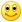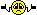# Thread: Riddle RAOK - WINNERS PICKED

1. d thanks2. Answer is D .. Thanks!

3.Originally Posted by JbirdI thought BEDMAS was just used when there was brackets involved in the equation?Nope, BEDMAS is the order used in any mathematical equation.

4. D
Thanks for the opportunity!

5. d

6. d

7. Yea I gotta go with E....none of the above8. d)9. Anything times zero is zero, no matter how pretty you make the equation10.Originally Posted by KrazEAnything times zero is zero, no matter how pretty you make the equationCorrect so 2x0=0 but when you add and subtract to a zero the answer will vary!
Using BEDMAS which is the proper way, would result in the answer not
Being zero!

11. i am going with d

12. d.

13.Originally Posted by PinkypieCorrect so 2x0=0 but when you add and subtract to a zero the answer will vary!
Using BEDMAS which is the proper way, would result in the answer not
Being zero!

Well, if we use PEMDAS (Parentheses, Exponents, Multiplication, Division, Addition, Subtraction), and knowing that multiplication and division occur at the same time in an equation but before addition or subtraction AND there are no parentheses surrounding the final segment of 2x0 then..

2+2+2+2+2+2+2+2+2+2+2+2-2+2x0 = ?

(e) None of the above

Perhaps she accidentally missed adding something more to the equation?

14. Brackets, Multiplication or Division then Addition or Subtraction totals 22

15. D , I might be bad at math but I do know that!!